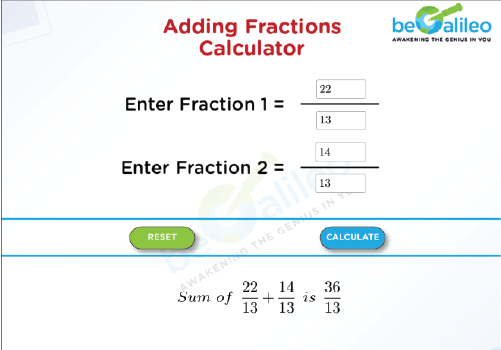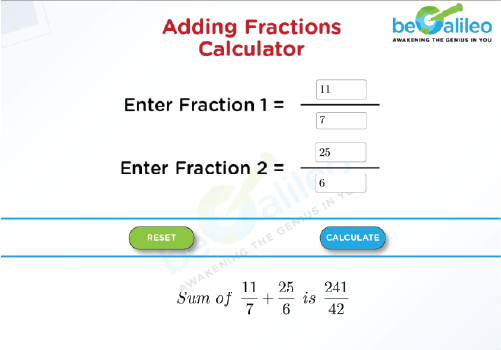>### Master Math with 1:1 Online Classes. Learn from Experts!Adding Fractions Calculator is a tool that will help us in calculating the sum of two fractions.
It can be applied to all forms of fractions, including proper, improper, and mixed fractions.

Adding Fractions Calculator is time saving and reduces the errors of human calculation as it involves various steps.

## How to add the given fractions using the Adding Fractions Calculator?

Step 1 : Enter the numerator and denominator of the first number in Fraction 1.

Step 2 : Enter the numerator and denominator of the second number in Fraction 2.

Step 3 : If you click “CALCULATE”, you will get their sum in its simplest form.

Step 4 : Further, click the “RESET” button to enter the next set of fractions.

## How do we add fractions?

Let us take two fractions \frac{a}{b}and \frac{c}{b}.

Sum = \frac{a+c}{b}

Add the numerator to the numerator, and the denominator will remain constant.

The denominator impacts fraction addition. When adding fractions, there are two possibilities, depending on whether the denominator is the same or different.

The group of two or more fractions having the same denominator is called like fractions.

The group of two or more fractions having the different denominators is called unlike fractions.

### Case 1: Adding like fractions.

If the denominators of the given two fractions are the same, we can add the numerator directly to the numerator while keeping their denominator in common.

\frac{4}{5}+\frac{7}{5}=\frac{(4+7)}{5}
=\frac{11}{5}

### Case 2: Adding unlike fractions.

We should do several steps to add unlike fractions.

• First, we must transform the unlike fraction into the like fraction in order to find their sum.
• For the conversion, the denominators should be made common.
• We must find the Least Common Multiple (LCM) of the given denominators.
• The (LCM) will be used as the common denominator.
• For each fraction, multiply the numerator and denominator by the same multiple to get the LCM as the denominator.
• Once we have the common denominator, we can simply add the numerators to get the sum.

Example : Add the fractions : \frac{2}{4}+\frac{6}{3}

The given denominators are 4 and 3.
LCM of 4 and 3 is 12.
\frac{2}{4}=\frac{2\times3}{4\times3}=\frac{6}{12}
\frac{6}{3}=\frac{6\times4}{3\times4}=\frac{24}{12}
\frac{2}{4}+\frac{6}{3}=\frac{6}{12}+\frac{24}{12}=\frac{30}{12}

## Solved Examples

### Q1. Add the fractions: \frac{22}{13}+\frac{14}{13}

Solution :
Given fractions are like fractions.
So,
\frac{22}{13}+\frac{14}{13}=\frac{(22+14)}{13}
=\frac{36}{13}